# QuickSort

• Difficulty Level : Medium
• Last Updated : 27 Sep, 2022

Like Merge Sort, QuickSort is a Divide and Conquer algorithm. It picks an element as a pivot and partitions the given array around the picked pivot. There are many different versions of quickSort that pick pivot in different ways.

• Always pick the first element as a pivot.
• Always pick the last element as a pivot (implemented below)
• Pick a random element as a pivot.
• Pick median as the pivot.

The key process in quickSort is a partition(). The target of partitions is, given an array and an element x of an array as the pivot, put x at its correct position in a sorted array and put all smaller elements (smaller than x) before x, and put all greater elements (greater than x) after x. All this should be done in linear time.Partition Algorithm:

There can be many ways to do partition, following pseudo-code adopts the method given in the CLRS book. The logic is simple, we start from the leftmost element and keep track of the index of smaller (or equal to) elements as i. While traversing, if we find a smaller element, we swap the current element with arr[i]. Otherwise, we ignore the current element.

Pseudo Code for recursive QuickSort function:

/* low  –> Starting index,  high  –> Ending index */

quickSort(arr[], low, high) {

if (low < high) {

/* pi is partitioning index, arr[pi] is now at right place */

pi = partition(arr, low, high);

quickSort(arr, low, pi – 1);  // Before pi

quickSort(arr, pi + 1, high); // After pi

}

}

Pseudo code for partition()

/* This function takes last element as pivot, places the pivot element at its correct position in sorted array, and places all smaller (smaller than pivot) to left of pivot and all greater elements to right of pivot */

partition (arr[], low, high)
{
// pivot (Element to be placed at right position)
pivot = arr[high];

i = (low – 1)  // Index of smaller element and indicates the
// right position of pivot found so far

for (j = low; j <= high- 1; j++){

// If current element is smaller than the pivot
if (arr[j] < pivot){
i++;    // increment index of smaller element
swap arr[i] and arr[j]
}
}
swap arr[i + 1] and arr[high])
return (i + 1)
}

Illustration of partition() :

Consider: arr[] = {10, 80, 30, 90, 40, 50, 70}

• Indexes:  0   1   2   3   4   5   6
• low = 0, high =  6, pivot = arr[h] = 70
• Initialize index of smaller element, i = -1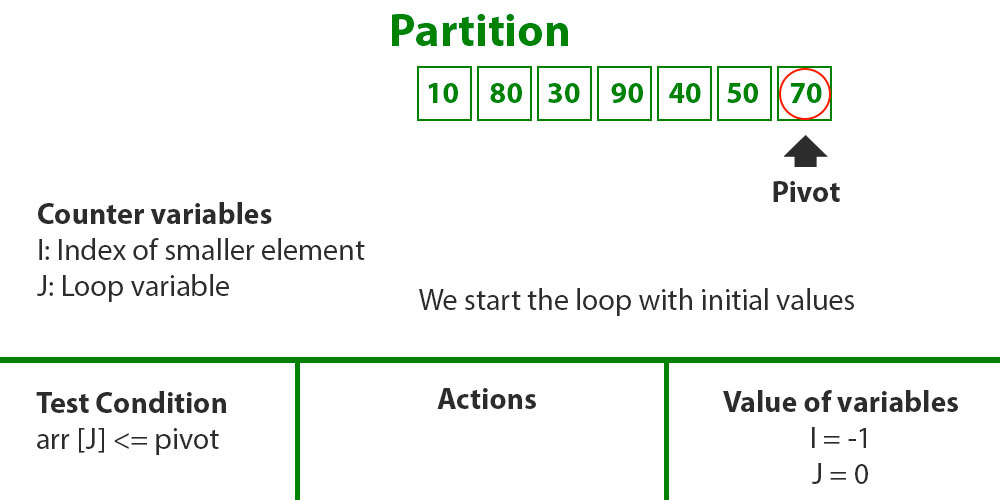• Traverse elements from j = low to high-1
• j = 0: Since arr[j] <= pivot, do i++ and swap(arr[i], arr[j])
• i = 0
• arr[] = {10, 80, 30, 90, 40, 50, 70} // No change as i and j are same
• j = 1: Since arr[j] > pivot, do nothing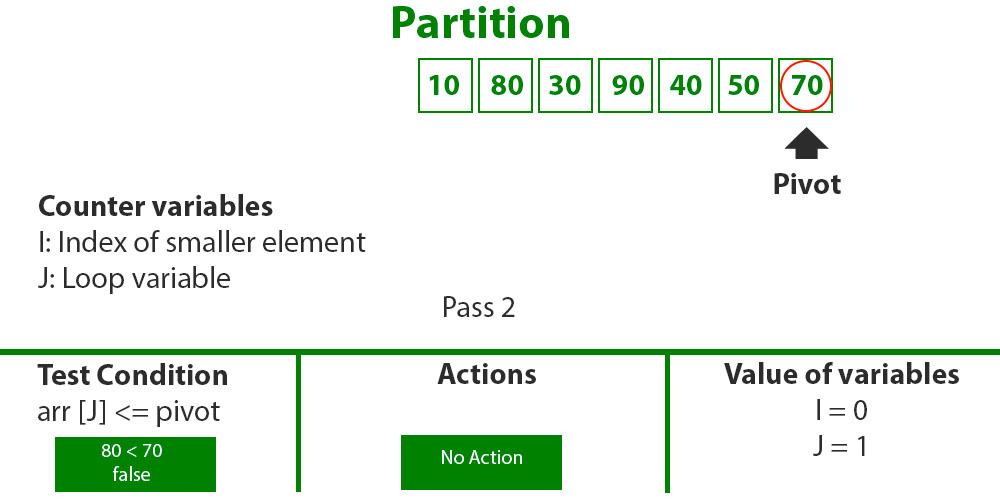• j = 2 : Since arr[j] <= pivot, do i++ and swap(arr[i], arr[j])
• i = 1
• arr[] = {10, 30, 80, 90, 40, 50, 70} // We swap 80 and 30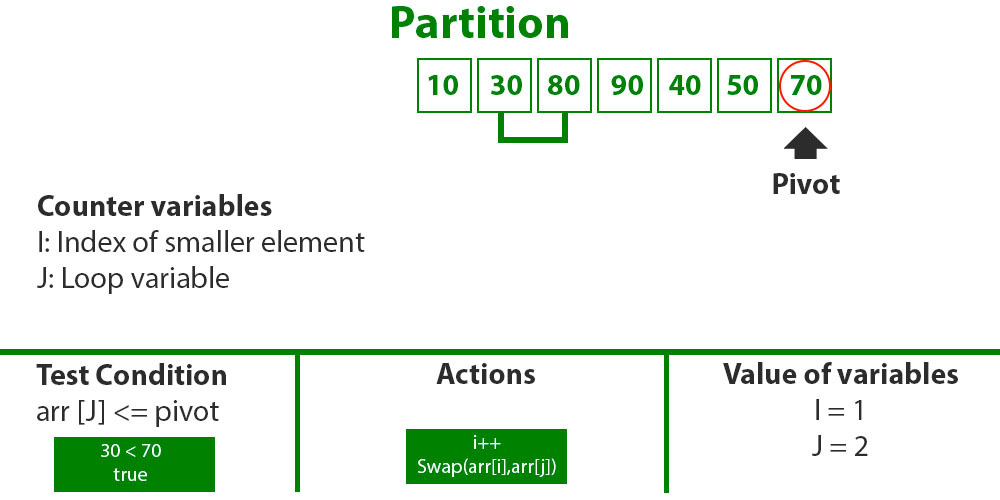• j = 3 : Since arr[j] > pivot, do nothing // No change in i and arr[]
• j = 4 : Since arr[j] <= pivot, do i++ and swap(arr[i], arr[j])
• i = 2
• arr[] = {10, 30, 40, 90, 80, 50, 70} // 80 and 40 Swapped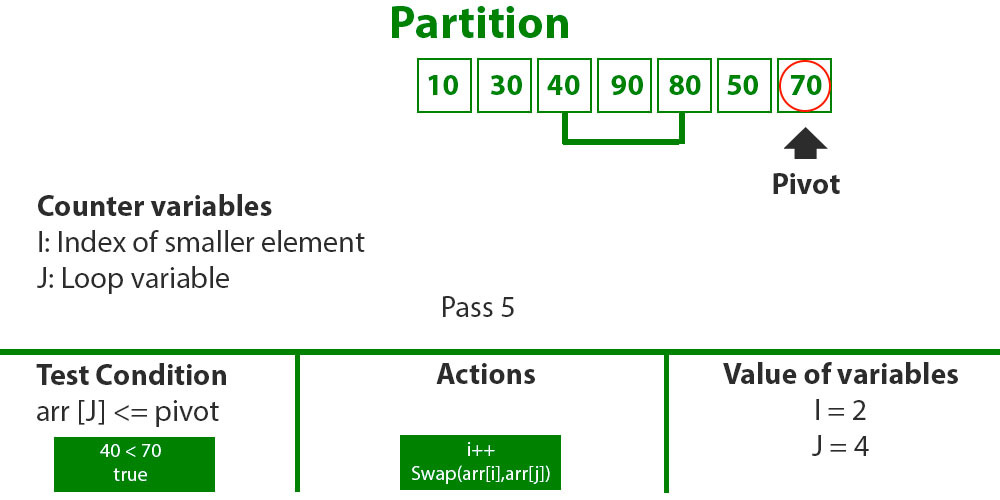• j = 5 : Since arr[j] <= pivot, do i++ and swap arr[i] with arr[j]
• i = 3
• arr[] = {10, 30, 40, 50, 80, 90, 70} // 90 and 50 Swapped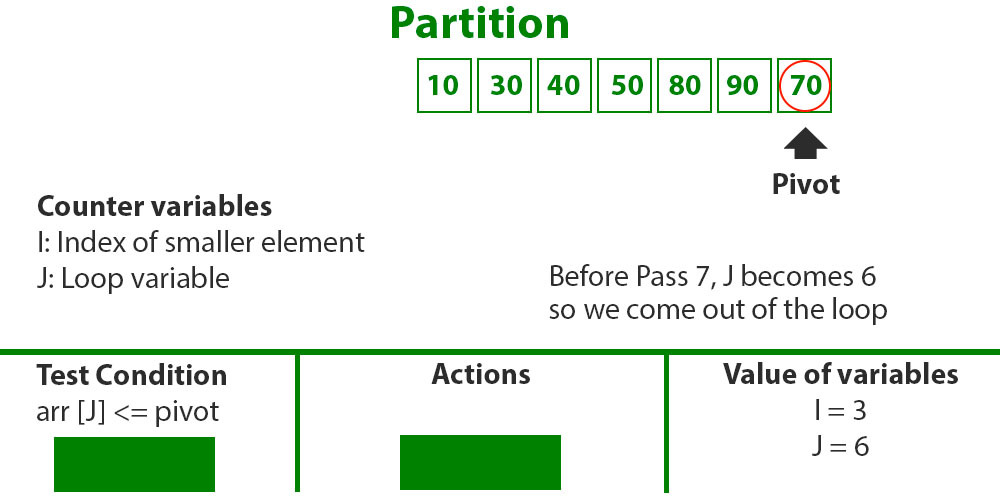• We come out of loop because j is now equal to high-1.
• Finally we place pivot at correct position by swapping arr[i+1] and arr[high] (or pivot)
• arr[] = {10, 30, 40, 50, 70, 90, 80} // 80 and 70 Swapped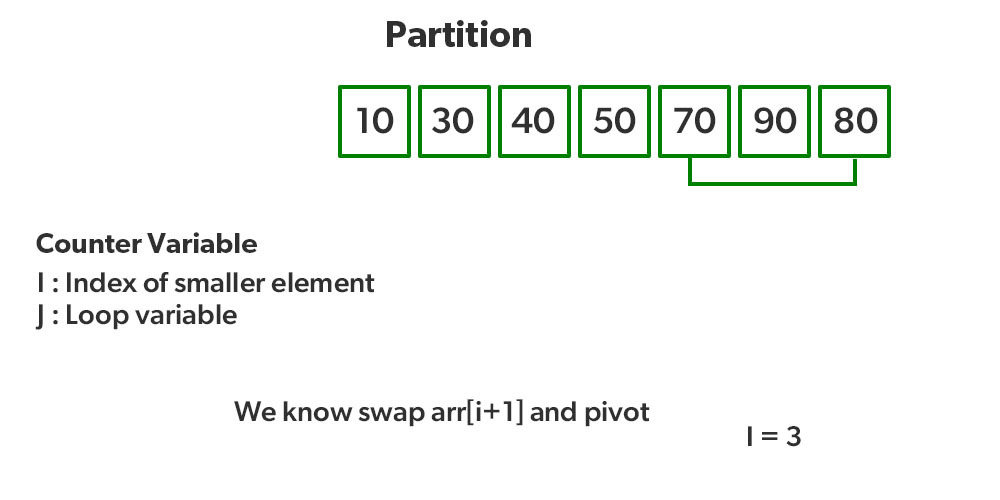• Now 70 is at its correct place. All elements smaller than 70 are before it and all elements greater than 70 are after it.
• Since quick sort is a recursive function, we call the partition function again at left and right partitions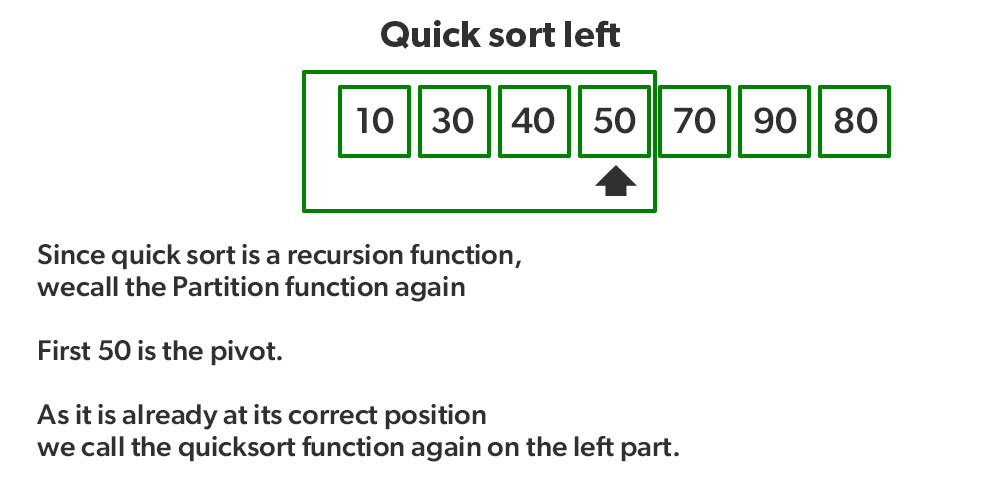• Again call function at right part and swap 80 and 90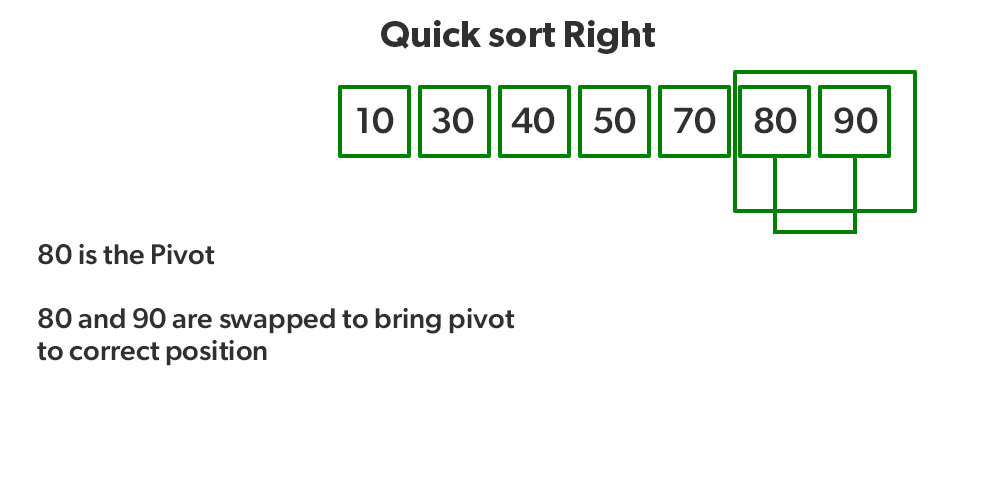Recommended PracticeImplementation:
Following are the implementations of QuickSort:

## C++14

 /* C++ implementation of QuickSort */#include using namespace std;  // A utility function to swap two elementsvoid swap(int* a, int* b){    int t = *a;    *a = *b;    *b = t;}  /* This function takes last element as pivot, placesthe pivot element at its correct position in sortedarray, and places all smaller (smaller than pivot)to left of pivot and all greater elements to rightof pivot */int partition(int arr[], int low, int high){    int pivot = arr[high]; // pivot    int i        = (low           - 1); // Index of smaller element and indicates                 // the right position of pivot found so far      for (int j = low; j <= high - 1; j++) {        // If current element is smaller than the pivot        if (arr[j] < pivot) {            i++; // increment index of smaller element            swap(&arr[i], &arr[j]);        }    }    swap(&arr[i + 1], &arr[high]);    return (i + 1);}  /* The main function that implements QuickSortarr[] --> Array to be sorted,low --> Starting index,high --> Ending index */void quickSort(int arr[], int low, int high){    if (low < high) {        /* pi is partitioning index, arr[p] is now        at right place */        int pi = partition(arr, low, high);          // Separately sort elements before        // partition and after partition        quickSort(arr, low, pi - 1);        quickSort(arr, pi + 1, high);    }}  /* Function to print an array */void printArray(int arr[], int size){    int i;    for (i = 0; i < size; i++)        cout << arr[i] << " ";    cout << endl;}  // Driver Codeint main(){    int arr[] = { 10, 7, 8, 9, 1, 5 };    int n = sizeof(arr) / sizeof(arr);    quickSort(arr, 0, n - 1);    cout << "Sorted array: \n";    printArray(arr, n);    return 0;}  // This code is contributed by rathbhupendra

## Java

 // Java implementation of QuickSortimport java.io.*;  class GFG {      // A utility function to swap two elements    static void swap(int[] arr, int i, int j)    {        int temp = arr[i];        arr[i] = arr[j];        arr[j] = temp;    }      /* This function takes last element as pivot, places       the pivot element at its correct position in sorted       array, and places all smaller (smaller than pivot)       to left of pivot and all greater elements to right       of pivot */    static int partition(int[] arr, int low, int high)    {          // pivot        int pivot = arr[high];          // Index of smaller element and        // indicates the right position        // of pivot found so far        int i = (low - 1);          for (int j = low; j <= high - 1; j++) {              // If current element is smaller            // than the pivot            if (arr[j] < pivot) {                  // Increment index of                // smaller element                i++;                swap(arr, i, j);            }        }        swap(arr, i + 1, high);        return (i + 1);    }      /* The main function that implements QuickSort              arr[] --> Array to be sorted,              low --> Starting index,              high --> Ending index     */    static void quickSort(int[] arr, int low, int high)    {        if (low < high) {              // pi is partitioning index, arr[p]            // is now at right place            int pi = partition(arr, low, high);              // Separately sort elements before            // partition and after partition            quickSort(arr, low, pi - 1);            quickSort(arr, pi + 1, high);        }    }      // Function to print an array    static void printArray(int[] arr, int size)    {        for (int i = 0; i < size; i++)            System.out.print(arr[i] + " ");          System.out.println();    }      // Driver Code    public static void main(String[] args)    {        int[] arr = { 10, 7, 8, 9, 1, 5 };        int n = arr.length;          quickSort(arr, 0, n - 1);        System.out.println("Sorted array: ");        printArray(arr, n);    }}  // This code is contributed by Ayush Choudhary

## Python3

 # Python3 implementation of QuickSort    # Function to find the partition positiondef partition(array, low, high):      # Choose the rightmost element as pivot    pivot = array[high]      # Pointer for greater element    i = low - 1      # Traverse through all elements    # compare each element with pivot    for j in range(low, high):        if array[j] <= pivot:            # If element smaller than pivot is found            # swap it with the greater element pointed by i            i = i + 1              # Swapping element at i with element at j            (array[i], array[j]) = (array[j], array[i])      # Swap the pivot element with     # e greater element specified by i    (array[i + 1], array[high]) = (array[high], array[i + 1])      # Return the position from where partition is done    return i + 1  # Function to perform quicksort    def quick_sort(array, low, high):    if low < high:          # Find pivot element such that        # element smaller than pivot are on the left        # element greater than pivot are on the right        pi = partition(array, low, high)          # Recursive call on the left of pivot        quick_sort(array, low, pi - 1)          # Recursive call on the right of pivot        quick_sort(array, pi + 1, high)    # Driver codearray = [10, 7, 8, 9, 1, 5]quick_sort(array, 0, len(array) - 1)  print(f'Sorted array: {array}')  # This code is contributed by Adnan Aliakbar

## C#

 // C# implementation of QuickSort  using System;  class GFG {      // A utility function to swap two elements    static void swap(int[] arr, int i, int j)    {        int temp = arr[i];        arr[i] = arr[j];        arr[j] = temp;    }      /* This function takes last element as pivot, places         the pivot element at its correct position in sorted         array, and places all smaller (smaller than pivot)         to left of pivot and all greater elements to right         of pivot */    static int partition(int[] arr, int low, int high)    {          // pivot        int pivot = arr[high];          // Index of smaller element and        // indicates the right position        // of pivot found so far        int i = (low - 1);          for (int j = low; j <= high - 1; j++) {              // If current element is smaller            // than the pivot            if (arr[j] < pivot) {                  // Increment index of                // smaller element                i++;                swap(arr, i, j);            }        }        swap(arr, i + 1, high);        return (i + 1);    }      /* The main function that implements QuickSort                arr[] --> Array to be sorted,                low --> Starting index,                high --> Ending index       */    static void quickSort(int[] arr, int low, int high)    {        if (low < high) {              // pi is partitioning index, arr[p]            // is now at right place            int pi = partition(arr, low, high);              // Separately sort elements before            // partition and after partition            quickSort(arr, low, pi - 1);            quickSort(arr, pi + 1, high);        }    }      // Function to print an array    static void printArray(int[] arr, int size)    {        for (int i = 0; i < size; i++)            Console.Write(arr[i] + " ");          Console.WriteLine();    }      // Driver Code    public static void Main()    {        int[] arr = { 10, 7, 8, 9, 1, 5 };        int n = arr.Length;          quickSort(arr, 0, n - 1);        Console.Write("Sorted array: ");        printArray(arr, n);    }}  // This code is contributed by gfgking

## Javascript

 

Output

Sorted array:
1 5 7 8 9 10 

### Hoare’s vs Lomuto Partition

Please note that the above implementation is Lomuto Partition. A more optimized implementation of QuickSort is Hoare’s partition which is more efficient than Lomuto’s partition scheme because it does three times less swaps on average.

### How to pick any element as pivot?

With one minor change to the above code, we can pick any element as pivot. For example, to make the first element as pivot, we can simply swap the first and last elements and then use the same code. Same thing can be done to pick any random element as a pivot

### Analysis of QuickSort

Time taken by QuickSort, in general, can be written as follows.

T(n) = T(k) + T(n-k-1) +(n)

The first two terms are for two recursive calls, the last term is for the partition process. k is the number of elements that are smaller than the pivot.
The time taken by QuickSort depends upon the input array and partition strategy. Following are three cases.

Worst Case:
The worst case occurs when the partition process always picks the greatest or smallest element as the pivot. If we consider the above partition strategy where the last element is always picked as a pivot, the worst case would occur when the array is already sorted in increasing or decreasing order. Following is recurrence for the worst case.

T(n) = T(0) + T(n-1) +(n)which is equivalent to  T(n) = T(n-1) +(n)

The solution to the above recurrence is(n2).

Best Case:
The best case occurs when the partition process always picks the middle element as the pivot. The following is recurrence for the best case.

T(n) = 2T(n/2) +(n)

The solution for the above recurrence is(nLogn). It can be solved using case 2 of Master Theorem.

Average Case:
To do average case analysis, we need to consider all possible permutation of array and calculate time taken by every permutation which doesn’t look easy
We can get an idea of average case by considering the case when partition puts O(n/9) elements in one set and O(9n/10) elements in other set. Following is recurrence for this case.

T(n) = T(n/9) + T(9n/10) +(n)

The solution of above recurrence is also O(nLogn):

Although the worst case time complexity of QuickSort is O(n2) which is more than many other sorting algorithms like Merge Sort and Heap Sort, QuickSort is faster in practice, because its inner loop can be efficiently implemented on most architectures, and in most real-world data. QuickSort can be implemented in different ways by changing the choice of pivot, so that the worst case rarely occurs for a given type of data. However, merge sort is generally considered better when data is huge and stored in external storage.

### Is QuickSort stable?

The default implementation is not stable. However any sorting algorithm can be made stable by considering indexes as comparison parameter.

### Is QuickSort In-place?

As per the broad definition of in-place algorithm it qualifies as an in-place sorting algorithm as it uses extra space only for storing recursive function calls but not for manipulating the input.

### What is 3-Way QuickSort?

In simple QuickSort algorithm, we select an element as pivot, partition the array around pivot and recur for subarrays on left and right of pivot.
Consider an array which has many redundant elements. For example, {1, 4, 2, 4, 2, 4, 1, 2, 4, 1, 2, 2, 2, 2, 4, 1, 4, 4, 4}. If 4 is picked as pivot in Simple QuickSort, we fix only one 4 and recursively process remaining occurrences. In 3 Way QuickSort, an array arr[l..r] is divided in 3 parts:

• arr[l..i] elements less than pivot.
• arr[i+1..j-1] elements equal to pivot.
• arr[j..r] elements greater than pivot.

See this for implementation.

### Can we implement QuickSort Iteratively?

Yes, please refer Iterative Quick Sort.

### Why Quick Sort is preferred over MergeSort for sorting Arrays ?

Quick Sort in its general form is an in-place sort (i.e. it doesn’t require any extra storage) whereas merge sort requires O(N) extra storage, N denoting the array size which may be quite expensive. Allocating and de-allocating the extra space used for merge sort increases the running time of the algorithm. Comparing average complexity we find that both type of sorts have O(NlogN) average complexity but the constants differ. For arrays, merge sort loses due to the use of extra O(N) storage space.
Most practical implementations of Quick Sort use randomized version. The randomized version has expected time complexity of O(nLogn). The worst case is possible in randomized version also, but worst case doesn’t occur for a particular pattern (like sorted array) and randomized Quick Sort works well in practice.
Quick Sort is also a cache friendly sorting algorithm as it has good locality of reference when used for arrays.
Quick Sort is also tail recursive, therefore tail call optimizations is done.

### Why MergeSort is preferred over QuickSort for Linked Lists ?

In case of linked lists the case is different mainly due to difference in memory allocation of arrays and linked lists. Unlike arrays, linked list nodes may not be adjacent in memory. Unlike array, in linked list, we can insert items in the middle in O(1) extra space and O(1) time. Therefore merge operation of merge sort can be implemented without extra space for linked lists.
In arrays, we can do random access as elements are continuous in memory. Let us say we have an integer (4-byte) array A and let the address of A be x then to access A[i], we can directly access the memory at (x + i*4). Unlike arrays, we can not do random access in linked list. Quick Sort requires a lot of this kind of access. In linked list to access i’th index, we have to travel each and every node from the head to i’th node as we don’t have continuous block of memory. Therefore, the overhead increases for quick sort. Merge sort accesses data sequentially and the need of random access is low.

### How to optimize QuickSort so that it takes O(Log n) extra space in worst case?

References:
http://en.wikipedia.org/wiki/Quicksort

Other Sorting Algorithms on GeeksforGeeks/GeeksQuiz:
Selection Sort, Bubble Sort, Insertion Sort, Merge Sort, Heap Sort, QuickSort, Radix Sort, Counting Sort, Bucket Sort, ShellSort, Comb Sort, Pigeonhole Sort
Please write comments if you find anything incorrect, or you want to share more information about the topic discussed above.

My Personal Notes arrow_drop_up